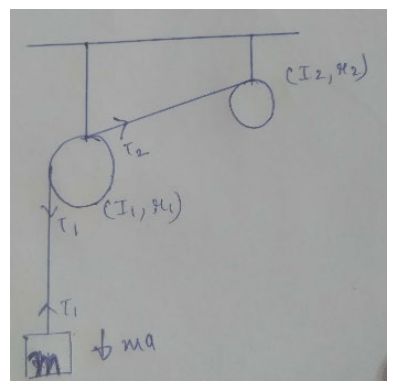# Solve the following :Question:

Suppose the smaller pulley of the previous problem has its radius $5.0 \mathrm{~cm}$ and moment of inertia $0.1 \mathrm{kgm}^{2}$. Find the tension in the part of the string joining the pulleys.

Solution:$m g-T_{1}=m a-\cdots-(i)$

$\left(T_{1}-T_{2}\right) r_{1}=I_{1} \alpha-----(i i)$

$T_{2} r_{2}=I_{2} \alpha-\cdots--(i i i)$

substitutingthe value of $T_{2}$ in equation (ii)

$\left(T_{1}-\frac{I_{2} \alpha}{r_{2}}\right) r_{1}=I_{1} \alpha$

$T_{1}-\frac{I_{2} \alpha}{r_{2}}=\frac{I_{1} \alpha}{r_{1}}$

$a s$

$a=\alpha r$

$T_{1}-\frac{I_{2} a}{r_{2}^{2}}=\frac{I_{1} a}{r^{2}}$

$T_{1}=\left(\frac{I_{2}}{r_{2}^{2}}+\frac{I_{1}}{r_{1}^{2}}\right) a$

Substitutingthe value of $T_{1}$ in equation 1

we get

$m g-\left(\frac{I_{2}}{r_{2}^{2}}+\frac{I_{1}}{r_{1}^{2}}\right) a=m a$

Onsubstituting the values

$\mathrm{a}=0.316 \mathrm{~ms}^{-2}$

$T_{2}=6.32 \mathrm{~N}$